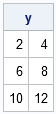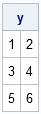The SAS/IML language secretly creates temporary variables. Most of the time programmers aren't even aware that the language does this. However, there is one situation where if you don't think carefully about temporary variables, your program will silently produce an error. And as every programmer knows, silent wrong numbers are the programmer's bane!

The situation in which you need to be careful is when you pass in an expression to a subroutine that changes the value of matrix. Recall that the SAS/IML language uses a pass-by-reference convention to pass parameters to functions and subroutines. This enables you to overwrite a parameter inside of a user-defined module. For example, the following subroutine doubles the values of all elements in the matrix passed into the function:

```proc iml; start Double(x); x = 2*x; finish;   y = {1 2, 3 4, 5 6}; run Double(y); print y;```Now suppose that you want to double ONLY the first column of y. This is where you need to be careful! If you have been programming all day and you are mentally tired, you might accidentally write the following statements:

```y = {1 2, 3 4, 5 6}; run Double( y[ ,1] ); print y;```As you see from the output, this statement does not change the elements of y. Furthermore, there is no error or warning that anything is wrong. If you hadn't printed the matrix, you might never have realized that the first column is unchanged.

You can avoid this error if you remember that SAS/IML creates temporary variables for expressions that involve subscripts and artihmetic operations. When the parser sees the call to the Double routine, it knows that it needs to send in a matrix as a parameter. It therefore creates a temporary matrix (let's call it _TEM001) for the expression y[ ,1]. It is this temporary matrix that is sent into the Double subroutine! Inside the subroutine, each value of the temporary matrix is doubled, but when the subroutine returns to the main program, the temporary matrix vanishes faster than a coin at a magician convention. Poof! It's gone! In particular, the temporary matrix is NOT copied into the first column of y. Consequently, the matrix y is unchanged.

There are two easy ways to double the second column correctly:

• Rewrite the Double subroutine as a function and call y[, 1] = Double( y[, 1] );
• Explicitly copy the first copy of y into a permanent matrix, call the Double function on that matrix, and copy back the result:

``` c1 = y[, 1]; run Double(c1); y[, 1] = c1;```

For more on temporary variables and a discussion of how SAS/IML evaluates arguments to functions, see p. 75–76 of Statistical Programming with SAS/IML software.

Share